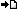RegisterMember LoginForgot Password ?? PHP , ASP , ASP.NET, VB.NET, C#, Java , jQuery , Android , iOS , Windows Phone

## Registered : 109,006

 HOME > PHP Manual > Comparison Operators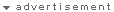## ลองใช้ค้นหาข้อมูล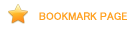## Comparison Operators

Comparison operators, as their name implies, allow you to compare two values. You may also be interested in viewing the type comparison tables, as they show examples of various type related comparisons.

Comparison Operators
Example Name Result
\$a == \$b Equal TRUE if \$a is equal to \$b.
\$a === \$b Identical TRUE if \$a is equal to \$b, and they are of the same type. (introduced in PHP 4)
\$a != \$b Not equal TRUE if \$a is not equal to \$b.
\$a <> \$b Not equal TRUE if \$a is not equal to \$b.
\$a !== \$b Not identical TRUE if \$a is not equal to \$b, or they are not of the same type. (introduced in PHP 4)
\$a < \$b Less than TRUE if \$a is strictly less than \$b.
\$a > \$b Greater than TRUE if \$a is strictly greater than \$b.
\$a <= \$b Less than or equal to TRUE if \$a is less than or equal to \$b.
\$a >= \$b Greater than or equal to TRUE if \$a is greater than or equal to \$b.

If you compare an integer with a string, the string is converted to a number. If you compare two numerical strings, they are compared as integers. These rules also apply to the switch statement.

``` <?phpvar_dump(0 == "a"); // 0 == 0 -> truevar_dump("1" == "01"); // 1 == 1 -> truevar_dump("1" == "1e0"); // 1 == 1 -> trueswitch ("a") {case 0:    echo "0";    break;case "a": // never reached because "a" is already matched with 0    echo "a";    break;}?> ```

For various types, comparison is done according to the following table (in order).

Comparison with Various Types
Type of Operand 1 Type of Operand 2 Result
null or string string Convert NULL to "", numerical or lexical comparison
bool or null anything Convert to bool, FALSE < TRUE
object object Built-in classes can define its own comparison, different classes are uncomparable, same class - compare properties the same way as arrays (PHP 4), PHP 5 has its own explanation
string, resource or number string, resource or number Translate strings and resources to numbers, usual math
array array Array with fewer members is smaller, if key from operand 1 is not found in operand 2 then arrays are uncomparable, otherwise - compare value by value (see following example)
array anything array is always greater
object anything object is always greater

Example #1 Transcription of standard array comparison

``` <?php// Arrays are compared like this with standard comparison operatorsfunction standard_array_compare(\$op1, \$op2){    if (count(\$op1) < count(\$op2)) {        return -1; // \$op1 < \$op2    } elseif (count(\$op1) > count(\$op2)) {        return 1; // \$op1 > \$op2    }    foreach (\$op1 as \$key => \$val) {        if (!array_key_exists(\$key, \$op2)) {            return null; // uncomparable        } elseif (\$val < \$op2[\$key]) {            return -1;        } elseif (\$val > \$op2[\$key]) {            return 1;        }    }    return 0; // \$op1 == \$op2}?> ```

See also strcasecmp(), strcmp(), Array operators, and the manual section on Types.

### Ternary Operator

Another conditional operator is the "?:" (or ternary) operator.

Example #2 Assigning a default value

``` <?php// Example usage for: Ternary Operator\$action = (empty(\$_POST['action'])) ? 'default' : \$_POST['action'];// The above is identical to this if/else statementif (empty(\$_POST['action'])) {    \$action = 'default';} else {    \$action = \$_POST['action'];}?> ```
The expression (expr1) ? (expr2) : (expr3) evaluates to expr2 if expr1 evaluates to TRUE, and expr3 if expr1 evaluates to FALSE.

Note: Please note that the ternary operator is a statement, and that it doesn't evaluate to a variable, but to the result of a statement. This is important to know if you want to return a variable by reference. The statement return \$var == 42 ? \$a : \$b; in a return-by-reference function will therefore not work and a warning is issued in later PHP versions.

Note: Is is recommended that you avoid "stacking" ternary expressions. PHP's behaviour when using more than one ternary operator within a single statement is non-obvious:

Example #3 Non-obvious Ternary Behaviour

``` <?php// on first glance, the following appears to output 'true'echo (true?'true':false?'t':'f');// however, the actual output of the above is 't'// this is because ternary expressions are evaluated from left to right// the following is a more obvious version of the same code as aboveecho ((true ? 'true' : 'false') ? 't' : 'f');// here, you can see that the first expression is evaluated to 'true', which// in turn evaluates to (bool)true, thus returning the true branch of the// second ternary expression.?> ```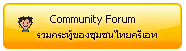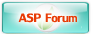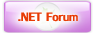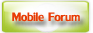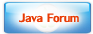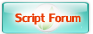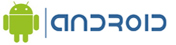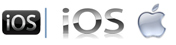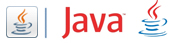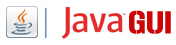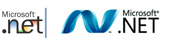Free Tutorial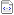สอน Google Maps Apiสอน Windows Serviceสอน Entity Frameworkสอน Androidสอน Java เขียน JavaJava GUI Swingสอน JSP (Web App)iOS (iPhone,iPad)Windows PhoneWindows AzureWindows StoreLaravel FrameworkYii PHP Frameworkสอน jQueryสอน jQuery กับ Ajaxสอน PHP OOP (Vdo)Ajax Tutorials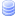SQL Tutorialsสอน SQL (Part 2)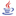JavaScript TutorialJavascript TipsVBScript TutorialVBScript ValidationMicrosoft Access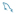MySQL Tutorials-- Stored ProcedureMariaDB Database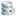SQL Server TutorialSQL Server 2005SQL Server 2008SQL Server 2012-- Stored ProcedureOracle Database-- Stored ProcedureSVN (Subversion)แนวทางการทำ SEOปรับแต่งเว็บให้โหลดเร็วสุดยอด Source Code V2.0แจ้งชำระเงิน/โอนเงินลองค้นหาสิ่งที่ต้องการLoad balance : Server 00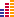© www.ThaiCreate.Com. 2003-2023 All Rights Reserved. ไทยครีเอทบริการ จัดทำดูแลแก้ไข Web Application ทุกรูปแบบ (PHP, .Net Application, VB.Net, C#) [Conditions Privacy Statement] ติดต่อโฆษณา 081-987-6107 อัตราราคา คลิกที่นี่